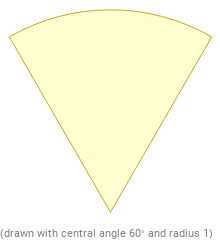Search IntMath
Close

# What Is the Sector of a Circle in Geometry?The sector of a circle in geometry is a simple shape made up of two lines and an arc. It is the portion of a circle that is enclosed by two radii of the circle and the arc that connects them. It is also known as a wedge of the circle. In this article, we will learn more about the concept of sector of a circle and how it relates to other elements of geometry.

## Understanding Arc, Radius, and Circumference

The arc of a circle is the curved line that makes up part of the sector of a circle. It is the line that connects the two radii and is measured in degrees. The radius of a circle is the line that connects the center of the circle to any point on the circumference. The circumference of a circle is the total length of the circle.

The arc length of a sector is equal to the central angle of the sector, multiplied by the circumference of the circle. The area of a sector is equal to one-half of the radius squared multiplied by the central angle of the sector.

## Understanding Chords

The chord of a circle is a straight line connecting two points on the circumference of the circle. The length of the chord is equal to twice the radius of the circle. The chord of a sector is the line that connects the two points on the circumference of the sector. The length of the chord of a sector is equal to twice the radius of the circle, multiplied by the central angle of the sector.

The area of a sector can also be determined by finding the area of the triangle formed by the two radii and the chord of the sector. This triangle is called the triangle of a sector and can be found by using the formula for the area of a triangle.

## Practice Problems

1. Find the area of a sector with a radius of 8 cm and a central angle of 60 degrees.

2. Find the length of the arc of a sector with a radius of 10 cm and a central angle of 120 degrees.

3. Find the length of the chord of a sector with a radius of 6 cm and a central angle of 45 degrees.

4. Find the area of a sector with a radius of 10 cm and a chord of length 16 cm.

5. Find the central angle of a sector with a radius of 4 cm and an arc length of 8p cm.

6. Find the length of the chord of a sector with a radius of 7 cm and an area of 56p cm2.

## Conclusion

The sector of a circle is the portion of the circle enclosed by two radii and the arc that connects them. It is important to understand the components of a sector, such as radius, circumference, arc, and chord, in order to correctly compute its area and arc length. With the right knowledge, you can easily calculate the area, arc length, or chord length of a sector.

We hope this article has helped you understand the concept of sector of a circle in geometry. If you have any doubts or questions, please feel free to ask in the comments section below.

## FAQ

### What is the formula to calculate the area of a sector of a circle?

The formula to calculate the area of a sector of a circle is A = (?/360) x pr2, where ? is the angle of the sector in degrees, and r is the radius of the circle.

### What is the formula to calculate the arc length of a sector of a circle?

The formula to calculate the arc length of a sector of a circle is L = (?/360) x 2pr, where ? is the angle of the sector in degrees, and r is the radius of the circle.

## Problem SolverThis tool combines the power of mathematical computation engine that excels at solving mathematical formulas with the power of GPT large language models to parse and generate natural language. This creates math problem solver thats more accurate than ChatGPT, more flexible than a calculator, and faster answers than a human tutor. Learn More.